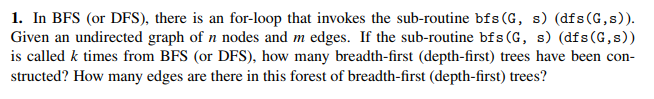# 1. In BFS (or DFS), there is an for-loop that invokes the sub-routine bfs (G, s) (dfs(G,s)) Given...

###### Question:1. In BFS (or DFS), there is an for-loop that invokes the sub-routine bfs (G, s) (dfs(G,s)) Given an undirected graph of n nodes and m edges. If the sub-routine bfs(G, s) (dfs (G,s)) is called k times from BFS (or DFS), how many breadth-first (depth-first) trees have been con- structed? How many edges are there in this forest of breadth-first (depth-first) trees?